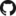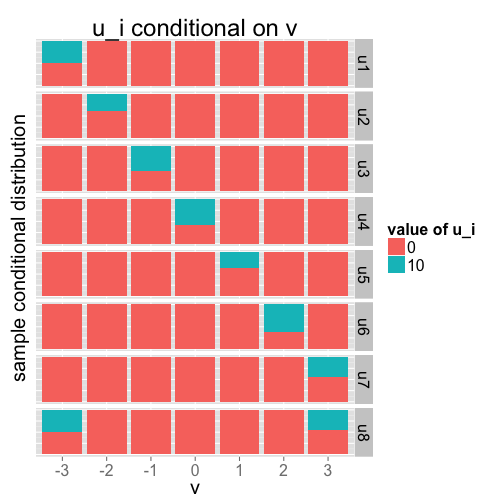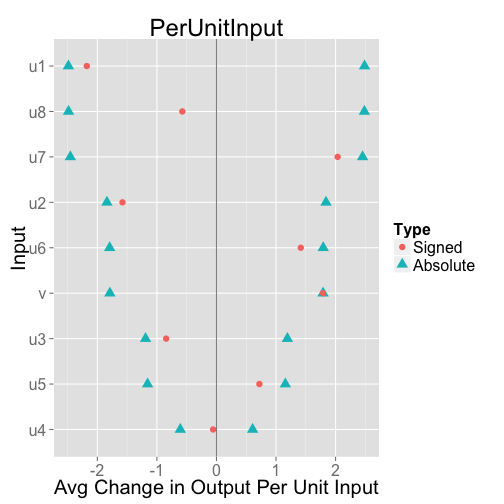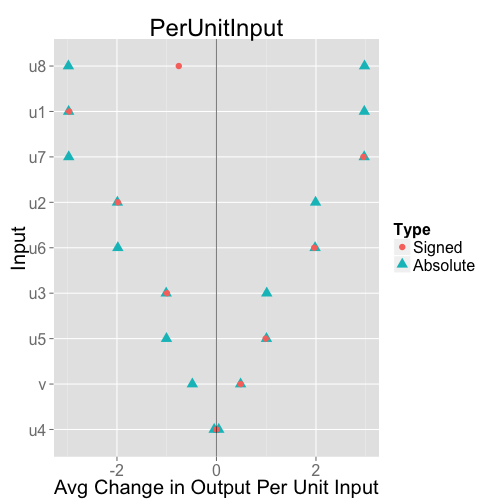# PredcompsHome

PDF Manual

Per Unit Input (original APC)

Impact (in units of output)

## Examples

Examples Overview

A fake logistic regression example predicting wine sales

A linear model with interactions

Loan defaults example

## More

As compared with Gelman & Pardoe 2007

Pairs & Weights

## APCs in a Synthetic Example of a Linear Model with Interactions

### Input Generation

This example will have 9 inputs: $$v$$ and $$u_1$$, $$u_2$$, …, $$u_8$$. The input $$v$$ is uniformly distributed between 7 values, $$-3, -2, \ldots, 2, 3$$. Each $$u$$ is mostly constant (at $$0$$) but at one value of $$v$$ (which is $$v=-3$$ for $$u_1$$, $$v=-2$$ for $$u_2$$, etc), $$u$$ can be either $$0$$ and $$10$$. The input $$u_8$$ can transition at two values of $$v$$: At either $$v=-3$$ or $$v=3$$, $$u_8$$ can be $$0$$ or $$10$$; otherwise, $$u_8$$ is 0. I'll construct the data and plot the relationship between the $$u$$'s and $$v$$.

N <- 200
vValues <- (-3):3
v <- sample(vValues, N, replace=TRUE)

df <- data.frame(v)
for (i in seq_along(vValues)) {
df[[paste0("u",i)]] <-
ifelse(v==vValues[i],
sample(c(0,10), N, replace=TRUE),  # u can be either 0 or 10 at one v value
rep(0, N)                          # u is always 0 at other ones
)
}

# u8 can transition at either v=-3 or v=3:
df$u8 <- ifelse(v %in% c(-3,3), sample(c(0,10), N, replace=TRUE), rep(0, N) )### Outcome Generation Each $$u$$ will have the same role in the model, which is $\mathbb{E}[y] = vu_1 + vu_2 + vu_3 + vu_4 + vu_5 + vu_6 + vu_7 + vu_8.$ Note that each $$u$$ has the same role in this function. Differences between their APCs can only arise from the correlation structure of the inputs in combination with this function, not from this function alone. For simplicity this demonstration, I'll assume that $$\mathbb{E}[y \mid v, u_1, \ldots, u_8]$$ is known rather than estimated. (Todo: Find a way to map these inputs/output to a possible story with meaningful features and outcome. That would make it more interesting, easier to follow, and maybe generate more insight.) outcomeGenerationFunction <- function(df) { with(df, v*u1 + v*u2 + v*u3 + v*u4 + v*u5 + v*u6 + v*u7 + v*u8) } df$y <- outcomeGenerationFunction(df)


### Computing and Plotting the APC

I will compute both the signed and absolute APC. My absolute APCs are different from anything discussed in the paper, but the idea is simple: Everywhere we would use a predictive difference, we use its absolute value instead. I believe that whenever we display the signed APC, we should display the absolute APC alongside it. Transitions for a given input may have a large expected absolute impact on the output variable, but with signs that cancel out. If that's happening in your model, it would be important to know.

Here are the APCs for this example, displayed in two different ways. The first shows signed and absolute APCs in separate charts. The second (my preference) shows both types of APC in the same chart. In this case, each absolute APC is plotted twice, once on the positive half of the APC axis and once on the negative half. This is necessary due to the symmetry between positive and negative numbers. The second version is my preference because it makes comparisons between signed and absolute APCs easier.

For example, we can more quickly notice in the second version that the signed APC for $$u_8$$ is small relative to its absolute APC. This is because $$u_8$$ transitions between 0 and 10 at either $$v=3$$ or $$v=-3$$. Either $$v$$ (combined with the $$u_8$$-transition) leads to a relatively large absolute predictive comparison, but with opposite signs depending on $$v$$.

inputVars <- c("v",paste0("u",1:8))
apcDF <- GetPredCompsDF(outcomeGenerationFunction, df, inputVars = inputVars)

## Working on: v
## Working on: u1
## Working on: u2
## Working on: u3
## Working on: u4
## Working on: u5
## Working on: u6
## Working on: u7
## Working on: u8

print(apcDF)

##    Input PerUnitInput.Signed PerUnitInput.Absolute Impact.Signed
## v      v             1.78983                1.7898        3.4938
## u1    u1            -2.17740                2.4860       -3.1153
## u2    u2            -1.57825                1.8392       -2.3853
## u3    u3            -0.84483                1.1910       -1.9541
## u4    u4            -0.05645                0.6074       -0.1239
## u5    u5             0.72105                1.1567        0.9354
## u6    u6             1.41604                1.7930        2.6609
## u7    u7             2.03453                2.4534        1.9358
## u8    u8            -0.57306                2.4837       -1.1852
##    Impact.Absolute
## v            3.494
## u1           3.557
## u2           2.780
## u3           2.755
## u4           1.333
## u5           1.501
## u6           3.369
## u7           2.334
## u8           5.137

PlotPredCompsDF(apcDF, variant="PerUnitInput")These APCs are just what we would expect from the setup of the synthetic examples. When $$u$$-transitions are at small $$v$$, the APCs are small due to the $$u$$/$$v$$ interaction effect (and vice versa). Even though we know the prediction function exactly, the APCs are a bit off due to errors in our estimates of the distribution of each input conditional on the others. We can mitigate that in this case by increasing the weight given to closer points when assigning weights based on the Mahalanobis distance. The weights are $$\frac{1}{\text{mahalanobisConstantTerm}+d}$$ where $$d$$ is the distance, so we can do this by decreasing $$\text{mahalanobisConstantTerm}$$:

apcDF <- GetPredCompsDF(outcomeGenerationFunction, df, inputVars, mahalanobisConstantTerm=.01)

## Working on: v
## Working on: u1
## Working on: u2
## Working on: u3
## Working on: u4
## Working on: u5
## Working on: u6
## Working on: u7
## Working on: u8

PlotPredCompsDF(apcDF, variant="PerUnitInput")In this case, the APCs are much closer to the correct values. Giving such extreme weight to pairs with small distance does not cause any problems in this example, but it would in more realistic examples. In applications, getting the weights right may be difficult.